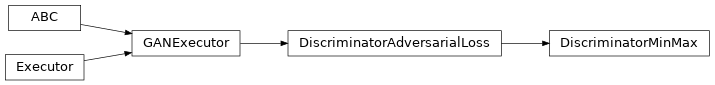# DiscriminatorMinMax¶

Inheritance Diagramclass ashpy.losses.gan.DiscriminatorMinMax(from_logits=True, label_smoothing=0.0)[source]

The min-max game played by the discriminator.

$L_{D} = - \frac{1}{2} E [\log(D(x)) + \log (1 - D(G(z))]$

Methods

 __init__([from_logits, label_smoothing]) Initialize Loss.

Attributes

 fn Return the Keras loss function to execute. global_batch_size Global batch size comprises the batch size for each cpu. weight Return the loss weight.
__init__(from_logits=True, label_smoothing=0.0)[source]

Initialize Loss.